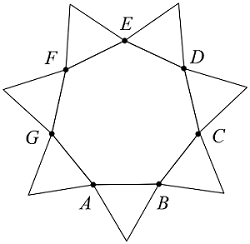# SAT Triangles-PerimeterIn the figure above, $ABCDEFG$ is a regular heptagon. To each of its sides is attached an equilateral triangle. If the perimeter of $ABCDEFG$ is 84, what is the perimeter of the star-shaped figure?

(A) $\ \ 84$
(B) $\ \ 96$
(C) $\ \ 144$
(D) $\ \ 168$
(E) $\ \ 216$

×